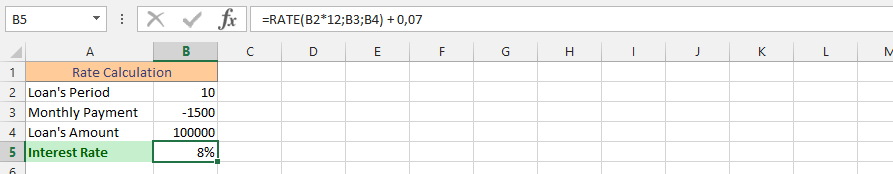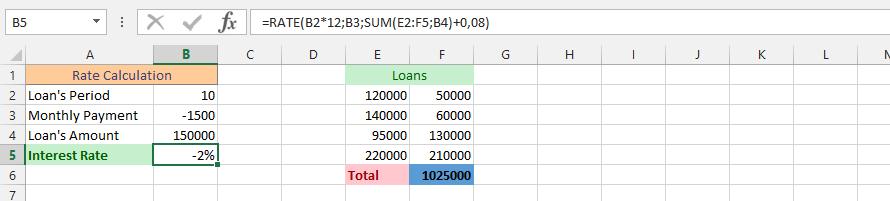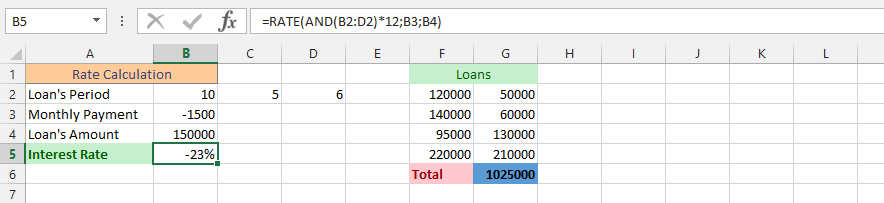### RATE Function in Excel and Different Formulas With It

In this lesson you will learn how to use RATE function in Excel.

Using RATE function you can calculate interest rates of investments or loans

### Rate function Parameters and Arguments

The syntax of the rate function is – =RATE(nper;pmt;pv;fv;type;guess)

and they are working together to return with the interest rate.

Nper: This is crucial to the function, and would be the total number of the payment periods in the annuity.

Pmt: This is required part of the RATE function. It is the payment that is made each period, and cannot be changed throughout the life of the annuity.

PVPresent value is required, and is the total amount that the future payments are currently worth

FV: This is the future value, and is optional. It is the cash balance that the user wishes to attain when the final payment has been made. If it is omitted, then the rate would assume it to be 0.

Type: This is optional, and can only be either 0 or 1. It is an indication of when the payments are due.

### Example 1 Interest rate of loan

The company offers installment sale. Installments are spread over 50 installments, the monthly repayment amount is \$15000. Price is \$1000000. What interest rate offers seller, per month and per year?

Syntax:

=RATE(50,-15000,1000000,0,0)

As you see you should type PMT with minus sign. You pay money off so it cause that you spend that money.

Answer: The result is the monthly interest rate: 1.07%. Yearly interest rate is 12*1.07% = 12.90%.

### Example 2 Interest rate for weekly installments

What is interest rate for \$10 000 loan. Weekly \$600 payments are made for 7 years. You have to pay at the beginning of week.

Syntax:

=RATE(7*52,-600,10000,,1)

Answer: The result is the weekly interest rate: 6.38%

### Example 3: Simple RATE Formula

We are in need of the interest rate that will make it possible to determine how much we could make from borrowing the client 100000.### Example 4: Complete Interest Rate Formula

We’d have to decide how much the interest rate would be, when our future goal is specific for the loan that we are lending the client. This is why we need to use a complete rate formula to find rate of the loan.### Example 5: What about the Previous Loan?

The client has a loan with the company before, and we would now like to know the interest rate, based on that, which is why we need to add that amount of money that the client has borrowed in the past.### Example 6: Rate plus 7%

We are adding 7 percent to the interest rate.### Example 7: SUM and Rate

The client has borrowed different amount of money from the company, and we have decided that once the loan has reached 500000, then the interest rate has to increase with 8 percent. This is why we are using the sum formula with the rate.### Example 8: Interest Rate of Payment

We are using the previous data to find the answers that we’d been looking for, and this is best acknowledged through both RATE and SUM. With exactly the same data as previous ones, we would like to acknowledge the interest rate of the data.### Example 9: AVERAGE and RATE

It is rare, but we are trying to evaluate a whole new scenario that will help us to find the crazy world that we have found ourselves in. Normally, we do not need the average, but this time, it has become necessary to find answers that help evaluate the scenario.### Example 10: RATE & AND Formula

The situation is quite flexible for how the payments would be made, and our number of total payment, which is why we are using both the RATE & AND formula.### Example 11: IF, SUM and RATE

We would like to find out the rate of the loan, after borrowing certain amount of money to the client. Normally speaking, we’d have a specific aim for rate, and we would like to know the rate after the loan amount reaches a specific amount of loan. This is why we are using IF, SUM and Rate formulas together to find answers.### Example 12: RATE, AND & SUM

We’d need the data to find out how the whole information would work out, and the only thing to do to find this answer, as the financial engineers say, is to use the rate, and & sum formulas simultaneously to find the answer that we’d need for the success of this acknowledgement.## Template

```Further reading: